2019-09-07 17:05:40 weixin_44225182 阅读数 1415
• ###### MATLAB图像处理

全面系统的学习MATLAB在图像处理中的应用

19896 人正在学习 去看看 魏伟

## 图像平移

I = imread('a1.jpg');
se = translate(strel(1), [50 140]);%将一个平面结构化元素分别向下和向右移动30个位置
J = imdilate(I,se);%利用膨胀函数平移图像
subplot(121);imshow(I), title('原图')
subplot(122), imshow(J), title('移动后的图像');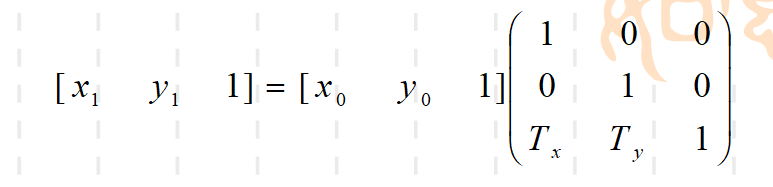I=rgb2gray(imread('a1.jpg'));%读入图片并转化为灰度图
subplot(1,2,1),imshow(I),title('原图');                   %建立窗口，显示灰度图I
[r,c]=size(I);                      %计算灰度图的大小，r表示行，c表示列，即通过size函数将灰度图I的行数存在矩阵的r中，列数存在矩阵的c中，这样就知道灰度图的大小是r×c
dst=zeros(r,c);                     %建立r×c的0矩阵（平移结果矩阵），初始化为零（黑色）
dx=50;                              %平移的x方向的距离，这里是竖直方向
dy=80;                              %平移的y方向的距离，这里是水平方向
tras=[1 0 dx;0 1 dy;0 0 1];         %平移变换矩阵
for i=1:r
for j=1:c
temp=[i;j;1];               %灰度图I要平移变换的点，这里用矩阵表示
temp=tras*temp;             %矩阵相乘，得到三行一列的矩阵temp，即平移后的矩阵
x=temp(1,1);                %把矩阵temp的第一行第一列的元素给x
y=temp(2,1);                %把矩阵temp的第二行第一列的元素给y
if(x>=1&&x<=r)&&(y>=1&&y<=c)%判断所变换后得到的点是否越界
dst(x,y)=I(i,j);        %得到平移结果矩阵，点(x,y)是由点(i,j)平移而来的，有对应关系
end
end
end
subplot(1,2,2),imshow(uint8(dst)),title('平移后');          %建立窗口，显示平移后的图t=imread('a1.jpg');

%分别提取三通道的矩阵
t_1=t(:,:,1)
t_2=t(:,:,2)
t_3=t(:,:,3)

%这里不能是[m,n]=size(t),我们是用三个二维矩阵合成一个三维图片（这里图片看出三维，毕竟有RGB）
[m,n,z]=size(t);

% 定义偏移量
dx=50;
dy=140;

%定义新矩阵 ，存储新坐标
r_1=zeros(m,n);
r_2=zeros(m,n);
r_3=zeros(m,n);

%martix 变换用的矩阵
martix=[1,0,dx;0,1,dy;0,0,1];

%坐标变换
for i=1:m
for j=1:n
tem=[i;j;1];
tem=martix*tem;
x=tem(1,1);
y=tem(2,1);
if(x>=1&&x<=m)&&(y>=1&&y<=n)
r_1(x,y)=t_1(i,j);
r_2(x,y)=t_2(i,j);
r_3(x,y)=t_3(i,j);
end
end
end

%得到三个新矩阵合成一张图片
rt=t
rt(:,:,1)=r_1;
rt(:,:,2)=r_2;
rt(:,:,3)=r_3;

%显示
subplot(1,2,1),imshow(t),title('原图');
subplot(1,2,2),imshow(rt),title('平移后');## 更多

2018-07-29 10:41:14 ywxk1314 阅读数 6475
• ###### MATLAB图像处理

全面系统的学习MATLAB在图像处理中的应用

19896 人正在学习 去看看 魏伟

1、代码如下：

（1）

close all;clear all;clc;  %关闭所有图形窗口，清除工作空间所有变量，清空命令行
a=50;b=50;
J1=move(I,a,b);
a=-50;b=50;
J2=move(I,a,b);
a=50;b=-50;
J3=move(I,a,b);
a=-50;b=-50;
J4=move(I,a,b);
set(0,'defaultFigurePosition',[100,100,1000,500]);
set(0,'defaultFigureColor',[1 1 1]);
figure(1);
subplot(121),imshow(J1),axis on;
subplot(122),imshow(J2),axis on;
figure(2);
subplot(121),imshow(J3),axis on;
subplot(122),imshow(J4),axis on;



（2）move函数代码：

function J = move( I,a,b )
%UNTITLED 此处显示有关此函数的摘要
%   此处显示详细说明
%a,b为平移量，I为原图像，J为平移后图像
[M,N,G]=size(I);
I=im2double(I);
J=ones(M,N,G);
for i=1:M
for j=1:N
if((i+a)>=1&&(i+a)<=M&&(j+b)>=1&&(j+b)<=N)  %判断平移后行列是否超过范围
J(i+a,j+b,:)=I(i,j,:);
end
end

end



2、运行效果如下：

（1）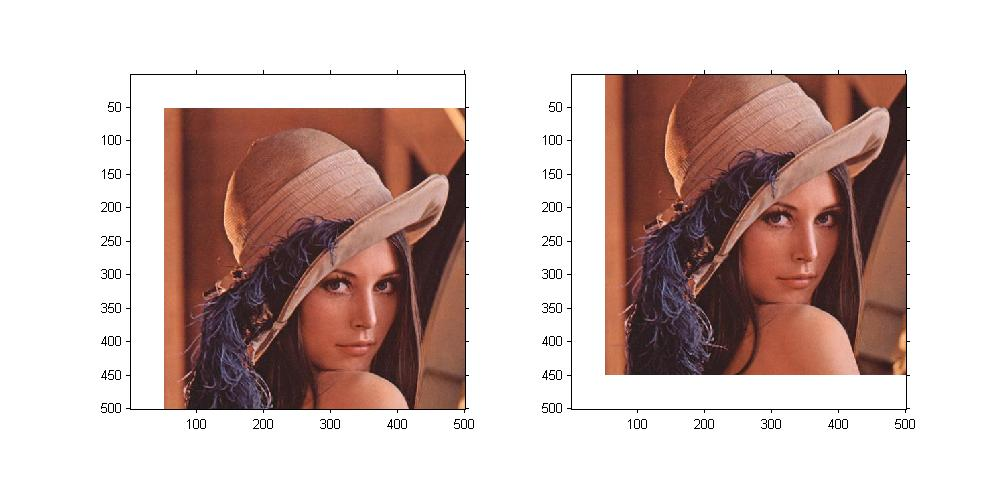（2）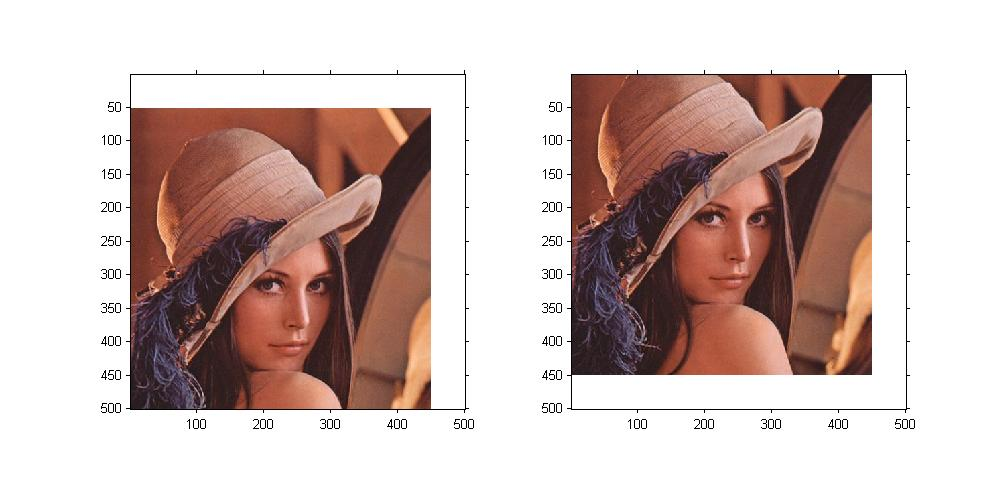2016-11-08 16:01:52 u012428169 阅读数 2644
• ###### MATLAB图像处理

全面系统的学习MATLAB在图像处理中的应用

19896 人正在学习 去看看 魏伟

#include <iostream>
#include <opencv2\core\core.hpp>
#include <opencv2\highgui\highgui.hpp>
#include <opencv2\imgproc\imgproc.hpp>

using namespace std;
using namespace cv;

/********************************************
void imMove(Mat img,Mat &dst,int x,int y)

Mat dst: 处理后得到的图像
int x： 水平右移距离
int y： 垂直下移距离

*********************************************/
void imMove(Mat img,Mat &dst, int x, int y)
{
int nHeight = img.rows;
int nWidth = img.cols;
int i, j;
if (x > nWidth || y > nHeight)
{
cout << "超出图片大小" << endl;
return;
}

for (i = 0; i < nHeight; i++)
{
for (j = 0; j < nWidth; j++)
{
if (i - x>0 && i - x<nHeight&&j - y>0 && j - y < nWidth)
dst.at<Vec3b>(i, j) = img.at<Vec3b>(i - x, j - y);
else
{
dst.at<Vec3b>(i, j) = 0;
dst.at<Vec3b>(i, j) = 0;
dst.at<Vec3b>(i, j) = 0;
}
}
}
}
int main()
{
Mat dst=img.clone();
imshow("原图", img);
//平移变换后
imMove(img,dst, 40, 60);
imshow("平移变换", dst);
waitKey(0);
return 0;
}2019-04-26 21:26:00 zaishuiyifangxym 阅读数 1315
• ###### MATLAB图像处理

全面系统的学习MATLAB在图像处理中的应用

19896 人正在学习 去看看 魏伟1 图像缩放- resize()

2 图像旋转- getRotationMatrix2D(), warpAffine()

3 图像翻转- flip()

4 图像平移- warpAffine()

# 1 图像缩放- resize()

result = cv2.resize(src, dsize[, result[. fx[, fy[, interpolation]]]])

src 表示原始图像；

dsize 表示缩放大小；

fx和fy 也可以表示缩放大小倍数，他们两个（dsize或fx/fy）设置一个即可实现图像缩放。例如：

（1）result = cv2.resize(src, (160,160))

（2）result = cv2.resize(src, None, fx=0.5, fy=0.5)

（1） cv2.resize(src, (200,100)) 设置的dsize是列数为200，行数为100

result = cv2.resize(src, (200,100))

# encoding:utf-8
import cv2
import numpy as np

# 读取图片

# 图像缩放
result = cv2.resize(src, (200,100))
print (result.shape)

# 显示图像
cv2.imshow("src", src)
cv2.imshow("result", result)

# 等待显示
cv2.waitKey(0)
cv2.destroyAllWindows()（2）可以获取 原始图像像素乘以缩放系数 进行图像变换；

result = cv2.resize(src, (int(cols * 0.6), int(rows * 1.2)))

# encoding:utf-8
import cv2
import numpy as np

# 读取图片
rows, cols = src.shape[:2]
print
rows, cols

# 图像缩放 dsize(列,行)
result = cv2.resize(src, (int(cols * 0.6), int(rows * 1.2)))

# 显示图像
cv2.imshow("src", src)
cv2.imshow("result", result)

# 等待显示
cv2.waitKey(0)
cv2.destroyAllWindows()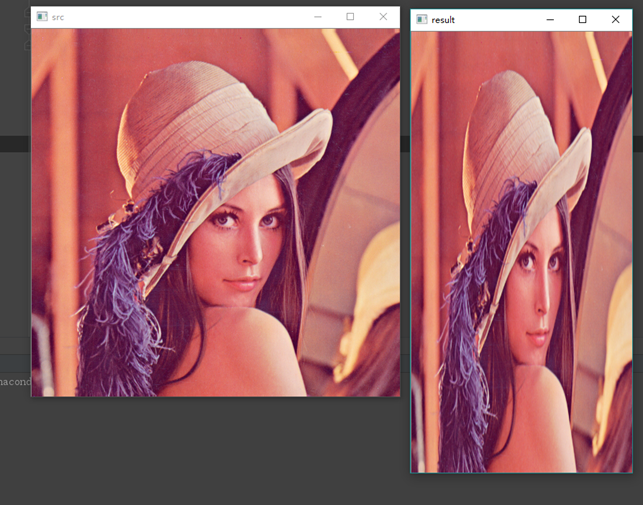（3）(fx,fy) 缩放倍数的方法对图像进行放大或缩小。

result = cv2.resize(src, None, fx=0.3, fy=0.3)

# encoding:utf-8
import cv2
import numpy as np

# 读取图片
rows, cols = src.shape[:2]
print
rows, cols

# 图像缩放
result = cv2.resize(src, None, fx=0.3, fy=0.3)

# 显示图像
cv2.imshow("src", src)
cv2.imshow("result", result)

# 等待显示
cv2.waitKey(0)
cv2.destroyAllWindows()# 2 图像旋转- getRotationMatrix2D(), warpAffine()

M = cv2.getRotationMatrix2D((cols/2, rows/2), 30, 1)

rotated = cv2.warpAffine(src, M, (cols, rows))

（1）旋转30度

# encoding:utf-8
import cv2
import numpy as np

# 读取图片

# 原图的高、宽 以及通道数
rows, cols, channel = src.shape

# 绕图像的中心旋转
# 参数：旋转中心 旋转度数 scale
M = cv2.getRotationMatrix2D((cols / 2, rows / 2), 30, 1)
# 参数：原始图像 旋转参数 元素图像宽高
rotated = cv2.warpAffine(src, M, (cols, rows))

# 显示图像
cv2.imshow("src", src)
cv2.imshow("rotated", rotated)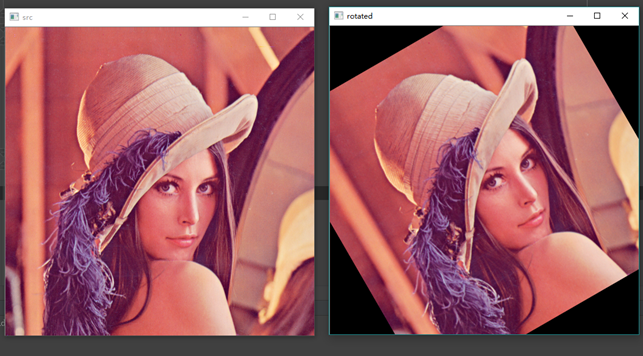（2）旋转90度

# encoding:utf-8
import cv2
import numpy as np

# 读取图片

# 原图的高、宽 以及通道数
rows, cols, channel = src.shape

# 绕图像的中心旋转
# 参数：旋转中心 旋转度数 scale
M = cv2.getRotationMatrix2D((cols / 2, rows / 2), 90, 1)
# 参数：原始图像 旋转参数 元素图像宽高
rotated = cv2.warpAffine(src, M, (cols, rows))

# 显示图像
cv2.imshow("src", src)
cv2.imshow("rotated", rotated)（3）旋转180度

# encoding:utf-8
import cv2
import numpy as np

# 读取图片

# 原图的高、宽 以及通道数
rows, cols, channel = src.shape

# 绕图像的中心旋转
# 参数：旋转中心 旋转度数 scale
M = cv2.getRotationMatrix2D((cols / 2, rows / 2), 180, 1)
# 参数：原始图像 旋转参数 元素图像宽高
rotated = cv2.warpAffine(src, M, (cols, rows))

# 显示图像
cv2.imshow("src", src)
cv2.imshow("rotated", rotated)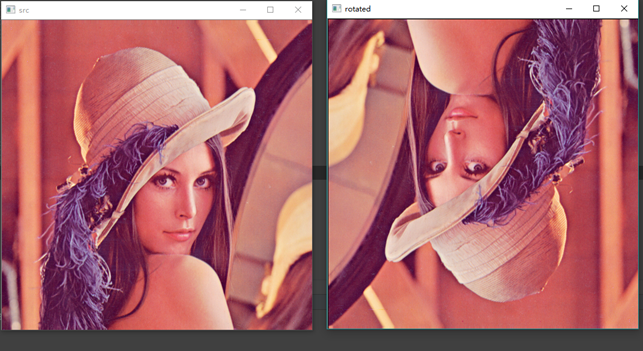# 3 图像翻转- flip()

dst = cv2.flip(src, flipCode)

src 表示原始图像；

flipCode 表示翻转方向，如果flipCode为0，则以X轴为对称轴翻转，如果fliipCode>0则以Y轴为对称轴翻转，如果flipCode<0则在X轴、Y轴方向同时翻转。

# encoding:utf-8
import cv2
import numpy as np
import matplotlib.pyplot as plt

# 读取图片
src = cv2.cvtColor(img, cv2.COLOR_BGR2RGB)

# 图像翻转
# 0以X轴为对称轴翻转 >0以Y轴为对称轴翻转 <0X轴Y轴翻转
img1 = cv2.flip(src, 0)
img2 = cv2.flip(src, 1)
img3 = cv2.flip(src, -1)

# 显示图形 （注意一个窗口多张图像的用法）
titles = ['Source', 'Image1', 'Image2', 'Image3']
images = [src, img1, img2, img3]
for i in range(4):
plt.subplot(2, 2, i + 1), plt.imshow(images[i], 'gray')
plt.title(titles[i])
plt.xticks([]), plt.yticks([])
plt.show()

# 等待显示
cv2.waitKey(0)
cv2.destroyAllWindows()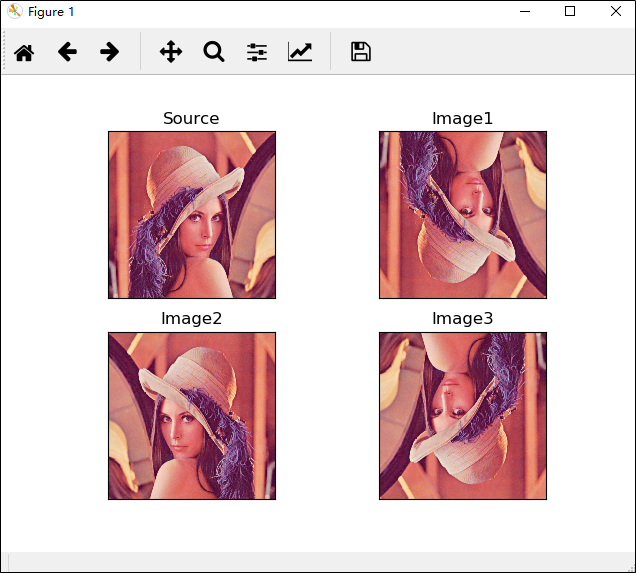# 4 图像平移- warpAffine()

M = np.float32([[1, 0, x], [0, 1, y]])

shifted = cv2.warpAffine(image, M, (image.shape, image.shape))

# encoding:utf-8
import cv2
import numpy as np
import matplotlib.pyplot as plt

# 读取图片
image = cv2.cvtColor(img, cv2.COLOR_BGR2RGB)

# 图像平移 下、上、右、左平移
M = np.float32([[1, 0, 0], [0, 1, 100]])
img1 = cv2.warpAffine(image, M, (image.shape, image.shape))

M = np.float32([[1, 0, 0], [0, 1, -100]])
img2 = cv2.warpAffine(image, M, (image.shape, image.shape))

M = np.float32([[1, 0, 100], [0, 1, 0]])
img3 = cv2.warpAffine(image, M, (image.shape, image.shape))

M = np.float32([[1, 0, -100], [0, 1, 0]])
img4 = cv2.warpAffine(image, M, (image.shape, image.shape))

# 显示图形
titles = ['Image1', 'Image2', 'Image3', 'Image4']
images = [img1, img2, img3, img4]
for i in range(4):
plt.subplot(2, 2, i + 1), plt.imshow(images[i], 'gray')
plt.title(titles[i])
plt.xticks([]), plt.yticks([])
plt.show()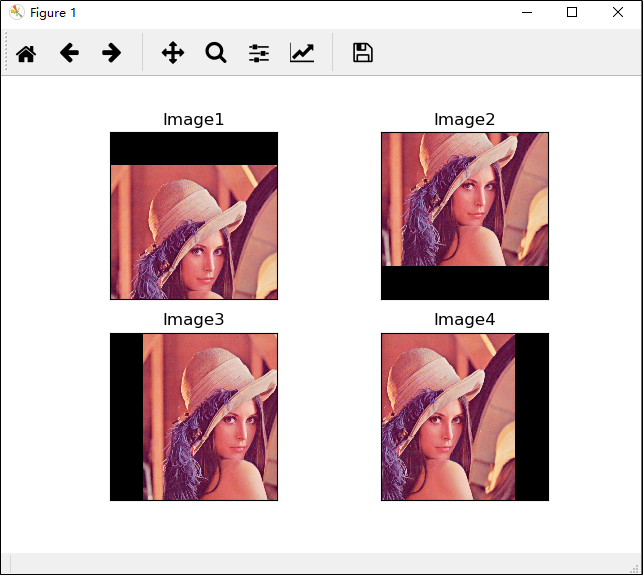# 参考资料

2013-07-09 20:03:29 liwuji930726 阅读数 2269
• ###### MATLAB图像处理

全面系统的学习MATLAB在图像处理中的应用

19896 人正在学习 去看看 魏伟

数字图像处理的主要两种方法：空域法和频域法

图像变换主要两种重要方式：图像几何变换（空间变换）和图像正交变换

图像几何变换是指原始图像按照需要进行大小、形状和位置的变化，属于空域法处理

图像位置变换主要包括图像平移、图像旋转、图像镜像、图像转置

1）将源图像保存到缓冲区，并记录下缓冲区的地址。

2）采用某种交互方式（如对话框），分别设定在水平和垂直方向上的平移量。

3）重新分配一个与源图像一样大小的临时缓冲区。

4）根据步骤2）中设定的平移量及源图像中每个像素点的坐标值，获得平移后各像素点的新坐标值，实现图像的平移，并且不显示已经移出源图区域的图像（如果超出源图的范围，则将该点的像素值统一设置为255（白色））

/*************************************************************************
* 函数名称：Translation(LPSTR lpSrcStartBits, long lWidth, long lHeight, long lXOffset,
long lYOffset,long lLineBytes,long lDstLineBytes)
* 函数参数:
*   LPSTR lpSrcStartBits，指向源DIB起始像素的指针
*   long lWidth，DIB图像的宽度
*   long lHeight，DIB图像的高度
*   long lXOffset,X方向偏移量
*   long lYOffset,Y方向偏移量
*   long lLineBytes，DIB图像的行字节数，为4的倍数
*   long lDstLineBytes，临时DIB图像的行字节数，为4的倍数
* 函数类型:BOOL
* 函数功能:该函数用来平移DIB图像
************************************************************************/
BOOL  Translation(LPSTR lpSrcStartBits, long lWidth, long lHeight,
long lXOffset, long lYOffset,long lLineBytes,long lDstLineBytes)

long i;                 //行循环变量
long j;                 //列循环变量
LPSTR lpSrcDIBBits; //指向源像素的指针
LPSTR lpDstDIBBits; //指向临时图像对应像素的指针
LPSTR lpDstStartBits; //指向临时图像对应像素的指针
HLOCAL hDstDIBBits; //临时图像句柄

hDstDIBBits= LocalAlloc(LHND, lWidth * lDstLineBytes);// 分配临时内存
lpDstStartBits= (char * )LocalLock(hDstDIBBits);// 锁定内存
if (hDstDIBBits== NULL)// 判断是否内存分配
return FALSE;// 分配内存失败
for(i = 0; i < lHeight; i++)// 行
{
for(j = 0; j < lWidth; j++) // 列
{
lpDstDIBBits=(char*)lpDstStartBits+lLineBytes*(lHeight-1-i)+j;// 指向新DIB第i行，第j个像素的指针
if( (j-lYOffset>= 0) && (j-lYOffset< lWidth) && // 像素在源DIB中的坐标j-lXOffset
(i-lXOffset>= 0) && (i-lXOffset < lHeight))// 判断是否在源图范围内
{
lpSrcDIBBits=(char *)lpSrcStartBits+lLineBytes*(lHeight-1-
(i-lXOffset))+(j-lYOffset);// 指向源DIB第i0行，第j0个像素的指针
*lpDstDIBBits= *lpSrcDIBBits;// 复制像素
}
else
{
*((unsigned char*)lpDstDIBBits) = 255;// 源图中没有的像素，赋为255
}
}
}
memcpy(lpSrcStartBits, lpDstStartBits, lLineBytes * lHeight);// 复制图像
LocalUnlock(hDstDIBBits);// 释放内存
LocalFree(hDstDIBBits);
return TRUE;
}

VC编程实现（基于VS2010）：